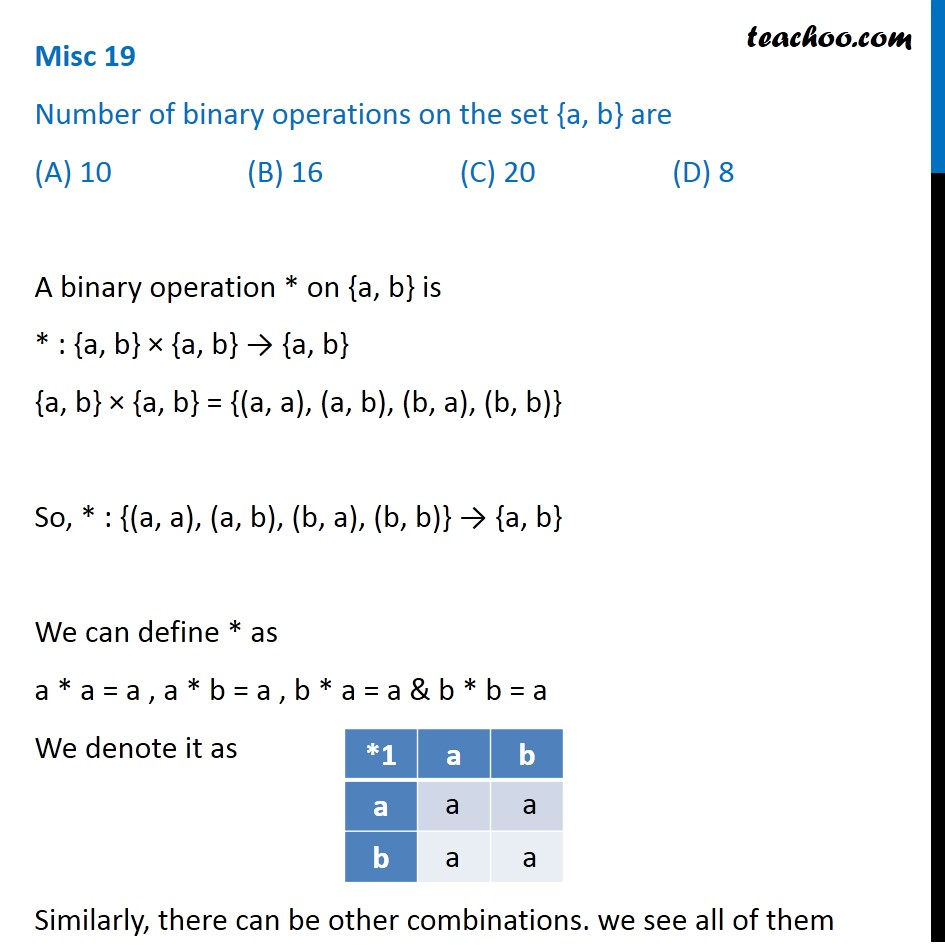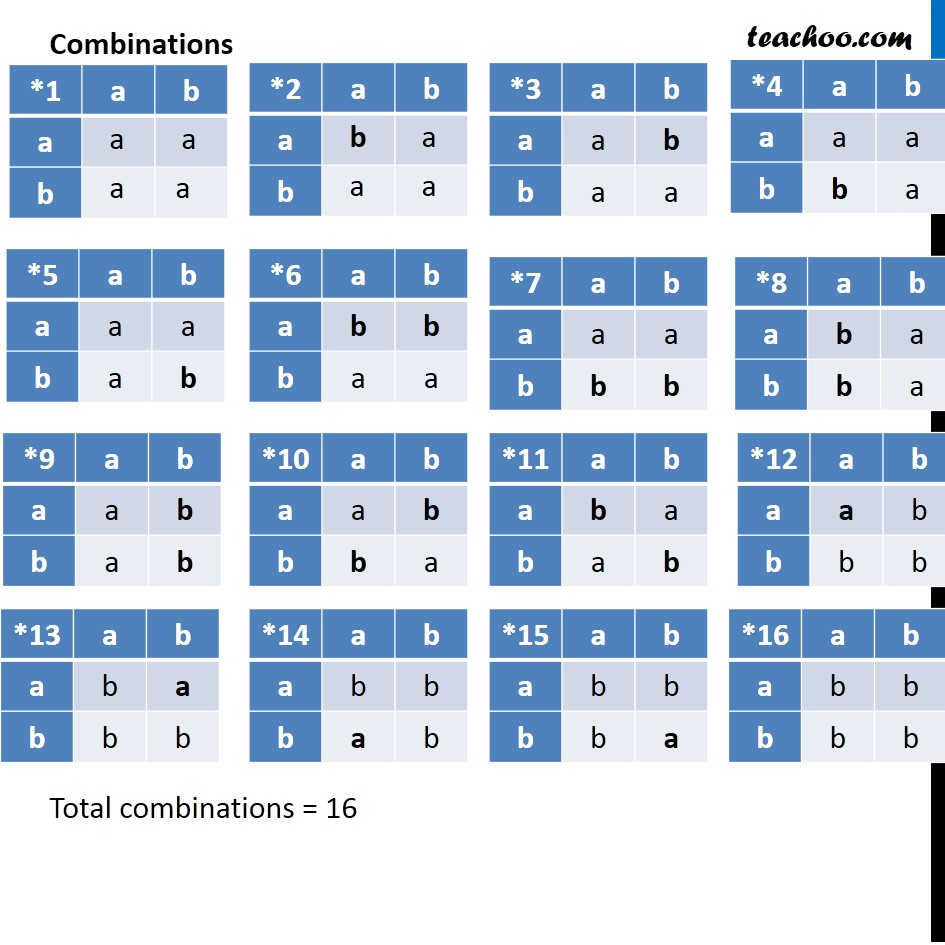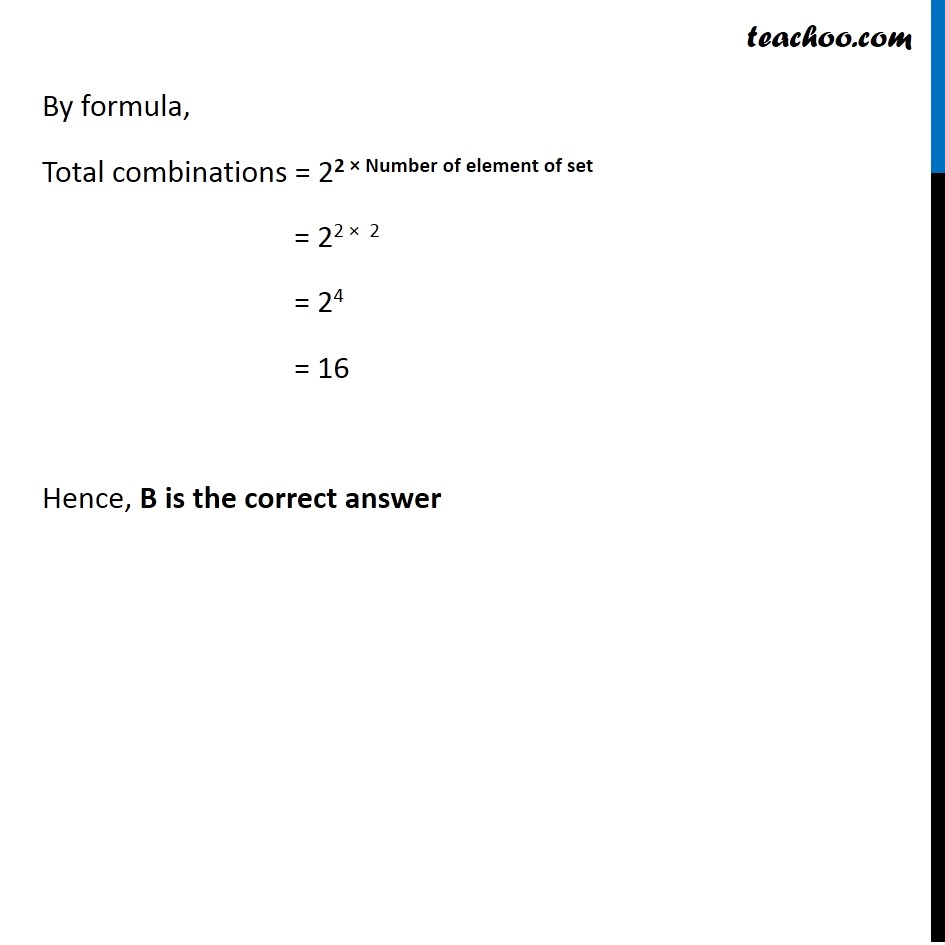Miscellaneous

Chapter 1 Class 12 Relation and Functions
Serial order wiseLearn in your speed, with individual attention - Teachoo Maths 1-on-1 Class

### Transcript

Misc 19 Number of binary operations on the set {a, b} are 10 (B) 16 (C) 20 (D) 8 A binary operation * on {a, b} is * : {a, b} × {a, b} → {a, b} {a, b} × {a, b} = {(a, a), (a, b), (b, a), (b, b)} So, * : {(a, a), (a, b), (b, a), (b, b)} → {a, b} We can define * as a * a = a , a * b = a , b * a = a & b * b = a We denote it as Similarly, there can be other combinations. we see all of them Combinations Total combinations = 16 By formula, Total combinations = 22 × Number of element of set = 22 × 2 = 24 = 16 Hence, B is the correct answer